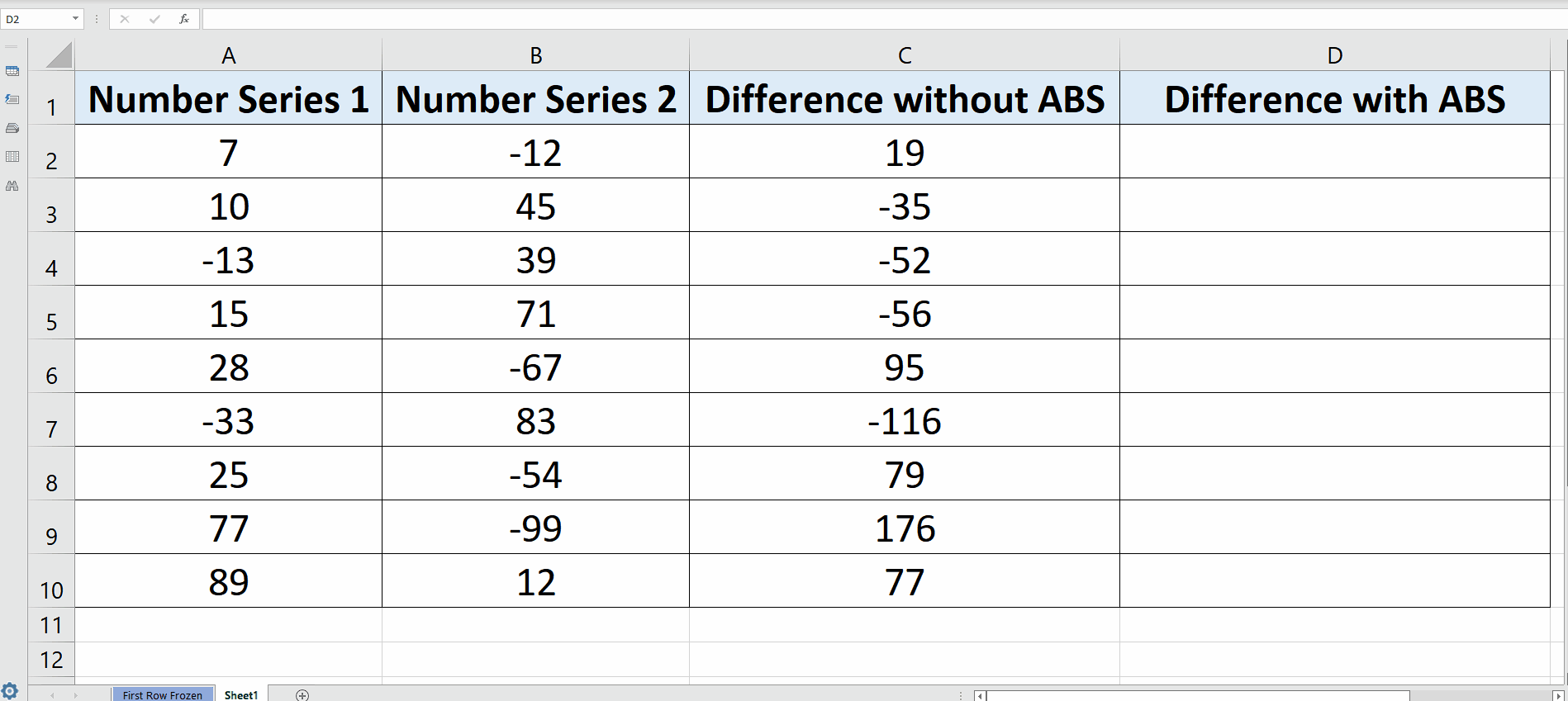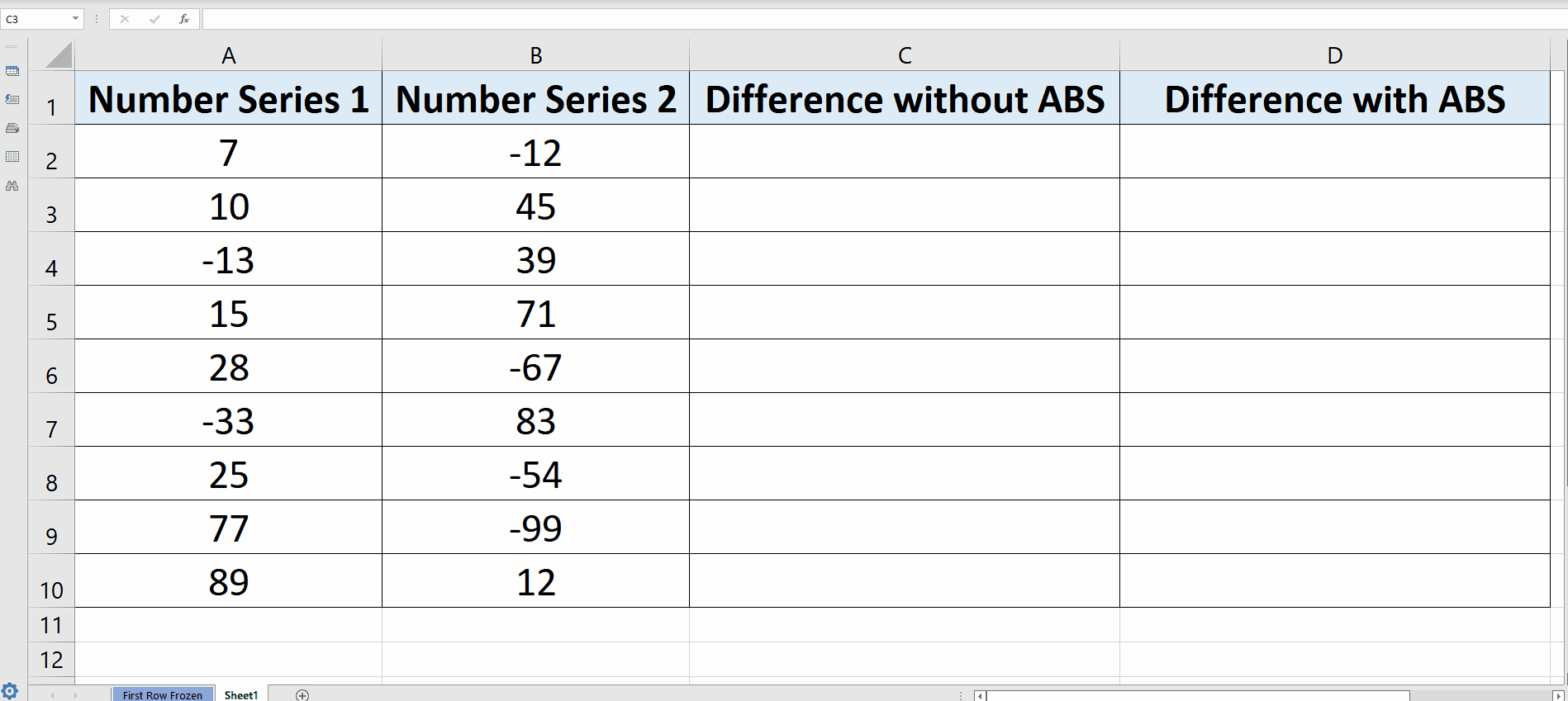# How to get Absolute Value in Excel

Excel provides a very simple method by using ABS function to get the absolute value of any single number or to convert the outcome of any complex calculation to absolute values. Let’s take a very simple example to understand how to achieve this. This is the data set that we have and we wish to calculate the difference of the numbers and let’s assume that we are only interested in finding out the value of the difference and not the sign of the difference. In this case we’ll take the difference both by using ABS and without using ABS to see what difference it makes.Sometimes while handling the numbers or quantities, we are only interested in finding out the magnitude of the quantities and we don’t care for their signs i.e. positive or negative. Technically speaking, the non-negative value of the number is known as the Absolute Value of that number.

### Step 1 – Take the difference without using ABS function– Choose the first cell where you wish to take the difference of Series A number and Series B numbers, in our case we will use C2.

– Use the following simple formula in that cell to calculate the difference and press enter key.
= A2 – B2

– The desired result will be displayed in this cell as soon as you press the enter key. Then drag down the formula by dragging the bottom right corner of C2 till the last data row as shown in the picture above.

So in this way the difference is calculated properly but with all signs i.e. negative signs.

Now let’s do the same thing but this time we will use the ABS function as well. Follow along these steps to do it.

### Step 2 – Take the difference using ABS function– Choose the first cell where you wish to take the difference of Series A number and Series B numbers, in our case we will use D2.

– Use the following simple formula in that cell to calculate the difference and press enter key.
= ABS(A2 – B2)

– The desired result will be displayed in this cell as soon as you press the enter key. Then drag down the formula by dragging the bottom right corner of D2 till the last data row as shown in the picture above.

This time when we calculated the difference of the same numbers we saw that ABS function returned us the same values but this time all values are without a negative sign. So this is how the ABS function works.